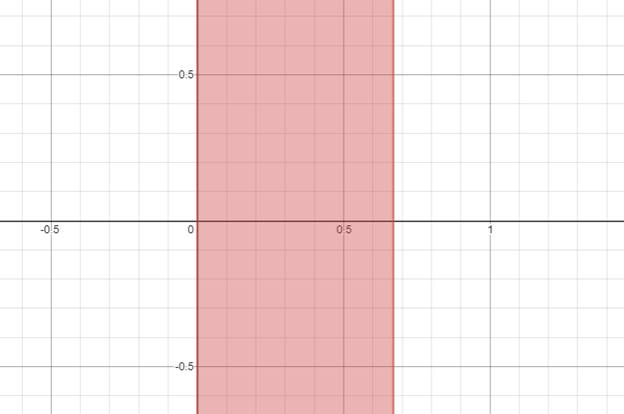# The solution of given nonlinear inequality equation and plot a graph for it.### Precalculus: Mathematics for Calcu...

6th Edition
Stewart + 5 others
Publisher: Cengage Learning
ISBN: 9780840068071### Precalculus: Mathematics for Calcu...

6th Edition
Stewart + 5 others
Publisher: Cengage Learning
ISBN: 9780840068071

#### Solutions

Chapter 1.7, Problem 38E
To determine

## The solution of given nonlinear inequality equation and plot a graph for it.

Expert Solution

0x0.67

### Explanation of Solution

Given:

Non-linear inequality equation

x(23x)0

Calculation,

Non-linear inequality equation,

x(23x)0x0or,(23x)03x2x23x0.67so,0x0.67

Now, graph of x(23x)0Conclusion:

Hence, the values of x lies between the 0 and 0.67.

### Have a homework question?

Subscribe to bartleby learn! Ask subject matter experts 30 homework questions each month. Plus, you’ll have access to millions of step-by-step textbook answers!